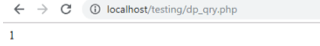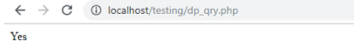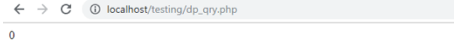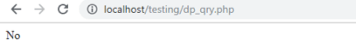Difference Between == and === in PHP# Difference between == and === in PHP

2770

Hey there,

Do you remember my previous blog demonstrating the difference between != and !==. Well, I was wondering if you can get confused between != and !==, you can very well also get confused between == and === in PHP, right!

Well, do not worry as this blog will be purely dedicated to resolving your concerns on the use of both == and === operators in PHP. In the meanwhile, you can also learn PHP online to get and insight into other operators in PHP.

Thus, without further ado, let us jump right into the differentiation of these two PHP operators.

== and ===: What's the Difference?

1. == Operator

Let us talk about the first operator, i.e. == or the equal operator, as we call it. The == operator is used to check whether the operand on both of the sides, right-hand side and left-hand side are equal in value or not. In other words, it checks whether both the operand holds the same value or not.

Confused? Let me explain this to you with the help of an example:

```if(0=="0")
{
echo "1";
}
else{
echo "0";
}```

This will generate and output as:Now, let us understand this with the help of another example:

```if(0==False)
{
echo "1";
}
else{
echo "0";
}```

In this code, the output for both of the functions will be 1 as 0==0 is true.Further, let us understand the use of == operator with a slightly simpler example:

```<?php
if("50" == 50) {
echo "YES";
} else {
echo "NO";
}
?>```

Here, the output will be "YES" as both of the operands hold the same value, i.e. 22.2. === Operator

On the other hand, ===, also known as the identical operator, is used to check for the similarity of operands, in terms of not only the value but also the type of the operand. This means that the operator will return the true values only if value as well as the type of both left-hand side and right-hand side operands, are equal.

Let us see the below example for === operator in PHP:

```<?php
if(0 === "0") {
echo "1";
} else {
echo "0";
}
?>```

On running the above code, the output will be generated as:Similarly, the following code below will also generate the same output:

```if(0===False)
{
echo "1";
}
else{
echo "0";
}```

After executing this code, the output will be 0, as the type of both operands is not the same. Although "0" is to string type, 0 is of numeric type.Now, let us look for the working of === by a similar example as we discussed for the equal operator:

```<?php
if("50" === 50) {
echo "Yes";
} else {
echo "No";
}
?>```

In the previous example with the equal (==) operator, this code generated the output as "Yes". However, this time, the code will give the output as "No".This is because the "50" under quotes is the string type and the 50 is the string type, which is not equal.

Conclusion

In a nutshell, the == operator is used to check for the equality of value between two operands and the === operator is used to check for the equality of value as well as the type of the operand. If you are preparing to be a PHP developer as your career path, you can also see our list of PHP Interview Questions which will help you prepare for the most likely questions asked during a PHP interview.

Meanwhile, if you think this blog is misleading or I have missed some point, you can reach out to me via your comment post below.

Till then, keep motivating and visiting my blog! Thank You!Share:## SEMRush FREE Trial – PRO Account for 14 Days

FREE Pro Account worth \$99.95 for 14 Days.

## Big Hosting Days

Upto 75@ OFF on Web Hosting

## Get a .COM for just \$6.98

Secure Domain for a Mini Price